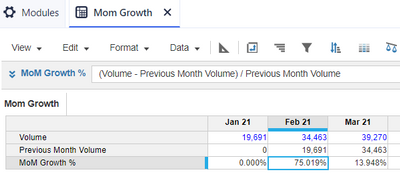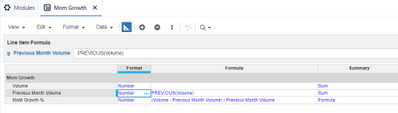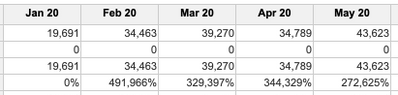# How to calculate Month to month Growth Rate?Hi all!

What is the way to calculate Month to Month Growth rate (from the beginning of the time scale)

if I have Sales Volume by month at monthly timescale?

I have:

(Volume by Month - PREVIOUS (Volume by Month)) /PREVIOUS (Volume by Month)* 100%

I wrote the formula but it doesn't match the math result (when I'm making calculation check)

Thank you.

Tagged:

•I like this formula but you might consider calculating the PREVIOUS(Volume) once as a line item then reference it. Also, you can just multiply by 100 (you can leave the percentage off). Lastly, the PREVIOUS function won't work too well on the first month since there's no previous for it to refer to. If the first month is important you can set up a separate module that contains just the  last month of the prior year. You'll then need to use a Boolean to identify the first month so you know to refer to the last month of the prior year.

Hope this helps!

•Hi @JoannaAn ,

Based on your table, i have done the below calculation.Add the logic if you want last month volume to be populated in the first months @JaredDolich  mentioned

Thanks

Akhtar

•Hi,

Formula looks correct, also you can optimize by the approach @JaredDolich  suggested. Can you share a couple of instance where it is giving wrong output.

Thanks

Abhay

•Break the formula out over several line items.

Volumes

Prior Volumes

Growth

You can also use an IF THEN ELSE statement to populate month 1 for Prior Volumes

=IF PREVIOUS(Volumes) <> 0  THEN PREVIOUS(Volumes) ELSE Volumes

This makes the assumption that this is the first month so closing volumes equal closing volumes. It Also assumes that every other month with have volumes greater than zero. If this is not the case then use @JaredDolich method of populating month 1.

Also, remove the * 100% and simply format the line item as a % in the blueprint.

•Thank you for the quick reply.

I just not sure that I'm using functions correctly.

And that's my formula is correct...

Because I'm getting different numbers by regular calculator..

So..what I would like to do is:

Growth (Feb20) = (34, 463 (Feb20) - 19,691(Jan20)) / 19,691(Jan20) * 100% = 75, 019 %

Growth (Mar20) = (39, 270 - 34, 463) / 34, 463 * 100% = 13, 94 %And here I have those huge numbers in the last row...

•Makes sure the summary method is formula not sum.
•Hi!

Yes, summary method was Sum..but when I'm trying to change it, it says:

PREVIOUS function cannot be used for summary time periods...

•Hi! Thank you for the reply.

I just posted my table with some numbers...

And more particular explanation if what I'm trying to do.. (Im thinking that may be I'm using wrong functions? not sure)

•Ok

You will need to use the ratio summary method.

Create two line items that calculate each side of the ratio and use these to populate the ratio settings.

The two new line items should both be set to sum.

•I think you're very close. @ChrisAHeathcote suggestion to turn off the SUM in summary method is a good one. Use Ratio or formula instead. I believe the reason you're seeing large percentages is because the values are being summed up the hierarchy. You got this! Good job on the formula!

•Ok! Thank you for your suggestion.

I will try to do so.

•Oh! Thank you @Akhtar.shahbaz

For now I only tried ratio, and Ratio didn't work for me for some reason...

And to make the Line item for the Previous months worked for me well.

(and it also allowed me to change sum - to formula that I couldn't do before)

I see now what @JaredDolich meant ...

Thanks to you,

and also to @JaredDolich  and @ChrisAHeathcote

I very much appreciate community help! 😃

Joanna.